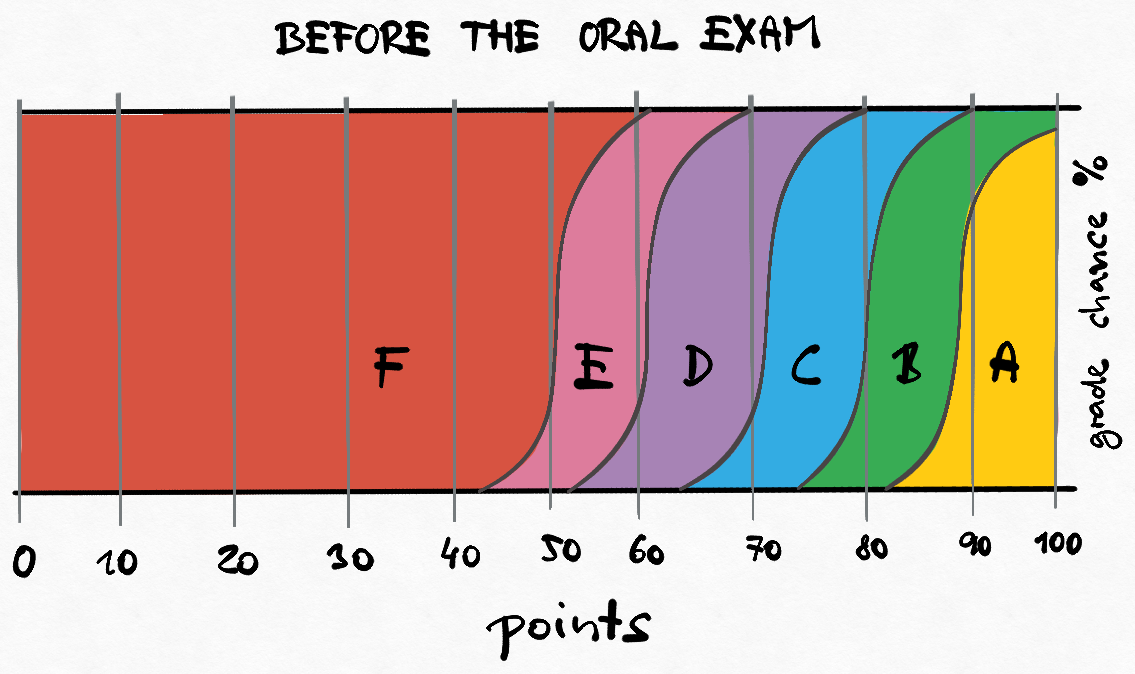Quick links: Schedule | Forum | BRUTE task submission system | Labs

# BE5B33RPZ -- Pattern Recognition and Machine Learning

## Summary

This course introduces statistical decision theory and surveys canonical and advanced classifiers such as perceptrons, AdaBoost, support vector machines, and neural nets.

## Basic info

Winter semester 2023/2024

Teaching: Jiří Matas (JM) matas@cmp.felk.cvut.cz, Ondřej Drbohlav (OD) drbohlav@cmp.felk.cvut.cz

Where and when: KN:E-301 at Building G, Karlovo namesti, Monday 16:15-17:45

Lectures are streamed live on YouTube at the time of lecture: online stream of the lecture room KN:E-301

Recorded lectures playlist

## Lecture plan 2023/2024

 Week Date Lect. Slides Topic Wiki Additional material 1 25.9. JM pdf Introduction. Basic notions. The Bayesian recognition problem Machine_learning Naive_Bayes_classifier some simple problems 2 2.10. OD pdf Non-Bayesian tasks Minimax 3 9.10. JM pdf Parameter estimation of probabilistic models. Maximum likelihood method Maximum_likelihood 4 16.10. JM pdf Nearest neighbour method. Non-parametric density estimation. K-nearest_neighbor_algorithm 5 13.10. JM pdf Logistic regression Logistic_regression 6 30.10. JM pdf Classifier training. Linear classifier. Perceptron. Linear_classifier Perceptron 7 6.11. JM pdf SVM classifier Support_vector_machine demo 8 13.11. JM pdf Adaboost learning Adaboost 9 20.11. JM pdf Neural networks. Backpropagation Artificial_neural_network 10 27.11. JM pdf Cluster analysis, k-means method K-means_clustering K-means++ 11 4.12. JM pdf EM (Expectation Maximization) algorithm. Expectation_maximization_algorithm Hoffmann,Bishop, Flach 12 11.12. JM pdf Feature selection and extraction. PCA, LDA. Principal_component_analysis Linear_discriminant_analysis Optimalizace (CZ): PCA slides, script 7.2 13 18.12. JM pdf Decision trees. Decision_tree Decision_tree_learning Rudin@MIT 14 8.1. JM Basic notions recapitulation, links between methods, answers to exam questions The lecture will not take place at the standard time, I'll be answering question by email or zoom link

## Assessment (zápočet)

Conditions for assessment are in the lab section.

## Exam

• Only students who receive all credits from the lab work and are granted the assessment (“zápočet”) can be examined.
• The labs contribute 50% to your final evaluation, the written part of the exam contributes 40% and the oral part 10%.
• A threshold for passing the exam is set, usually between 5-10 points (out of 40), depending on the complexity of the test.
• Your grade chances after the written exam test and before the oral exam are illustrated in the image below. Beware: The scheme is approximate only, but illustrates how the oral exam influences the final grade.
• The questions used in the test are available here (if one can solve these questions, one will likely do well in the exam)
• The oral starts approximately 2 hours after the end of the test, as soon as the tests are graded. Alternatively, the oral might take place the following day, if the number of tests to correct is high.
• The oral part is compulsory! Note that if during the oral examination it becomes clear that the student cannot explain how they obtained a result in the written exam, the examiner may re-assess the grading of the written exam.
• Example oral exam questions are available here.